Скачать презентацию Property-Based Testing a catalog of classroom examples Rex

cc977509ed9b4aaae0bd1590b907aa78.ppt

• Количество слайдов: 23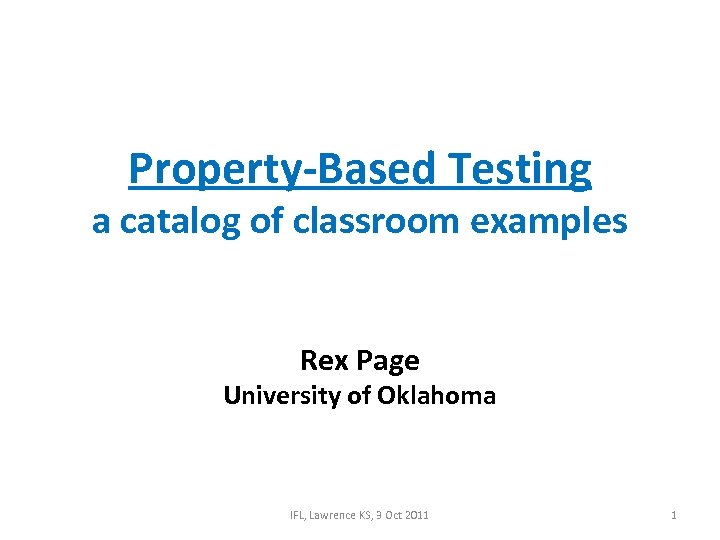Property-Based Testing a catalog of classroom examples Rex Page University of Oklahoma IFL, Lawrence KS, 3 Oct 2011 1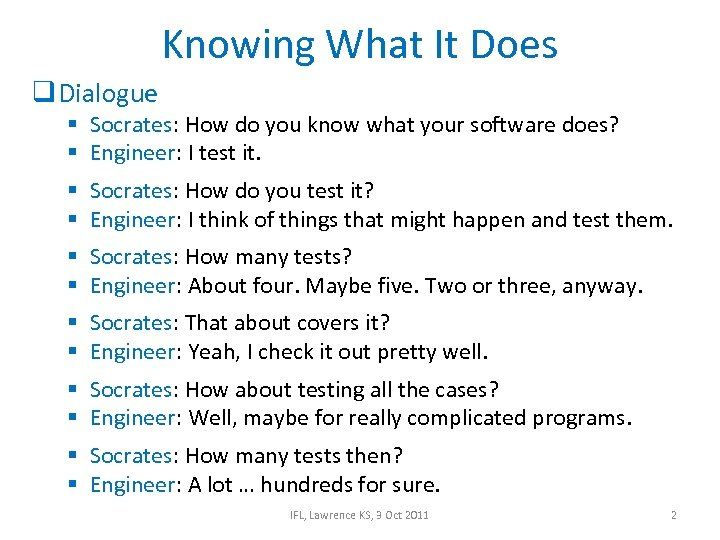Knowing What It Does q Dialogue § Socrates: How do you know what your software does? § Engineer: I test it. § Socrates: How do you test it? § Engineer: I think of things that might happen and test them. § Socrates: How many tests? § Engineer: About four. Maybe five. Two or three, anyway. § Socrates: That about covers it? § Engineer: Yeah, I check it out pretty well. § Socrates: How about testing all the cases? § Engineer: Well, maybe for really complicated programs. § Socrates: How many tests then? § Engineer: A lot … hundreds for sure. IFL, Lawrence KS, 3 Oct 2011 2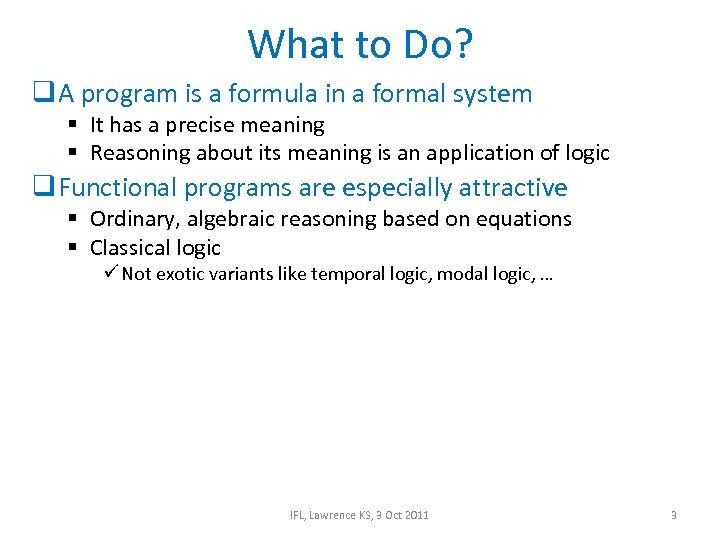What to Do? q A program is a formula in a formal system § It has a precise meaning § Reasoning about its meaning is an application of logic q Functional programs are especially attractive § Ordinary, algebraic reasoning based on equations § Classical logic ü Not exotic variants like temporal logic, modal logic, … IFL, Lawrence KS, 3 Oct 2011 3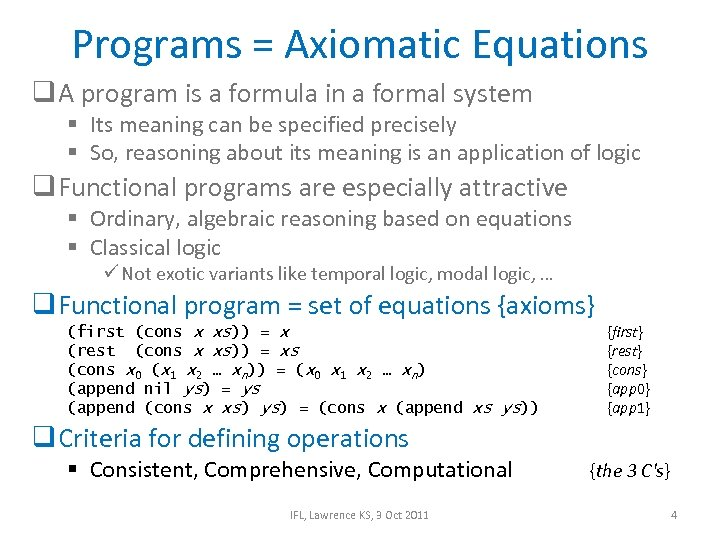Programs = Axiomatic Equations q A program is a formula in a formal system § Its meaning can be specified precisely § So, reasoning about its meaning is an application of logic q Functional programs are especially attractive § Ordinary, algebraic reasoning based on equations § Classical logic ü Not exotic variants like temporal logic, modal logic, … q Functional program = set of equations {axioms} (first (cons x xs)) = x (rest (cons x xs)) = xs (cons x 0 (x 1 x 2 … xn)) = (x 0 x 1 x 2 … xn) (append nil ys) = ys (append (cons x xs) ys) = (cons x (append xs ys)) {first} {rest} {cons} {app 0} {app 1} q Criteria for defining operations § Consistent, Comprehensive, Computational IFL, Lawrence KS, 3 Oct 2011 {the 3 C's} 4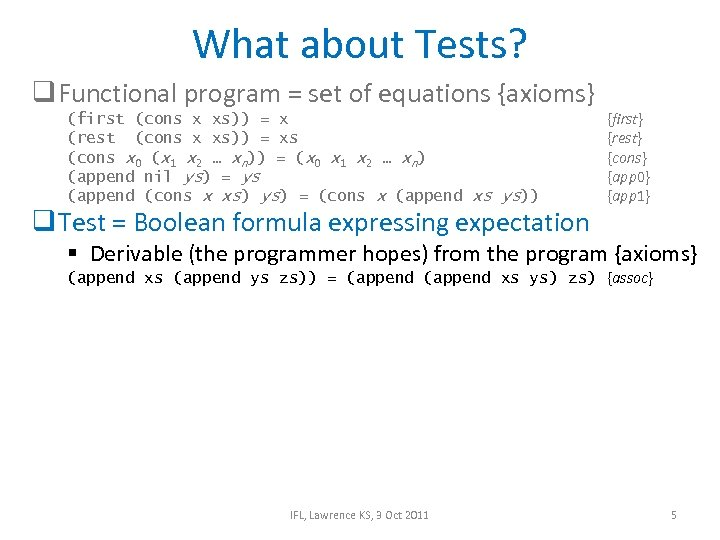What about Tests? q Functional program = set of equations {axioms} (first (cons x xs)) = x (rest (cons x xs)) = xs (cons x 0 (x 1 x 2 … xn)) = (x 0 x 1 x 2 … xn) (append nil ys) = ys (append (cons x xs) ys) = (cons x (append xs ys)) q Test = Boolean formula expressing expectation {first} {rest} {cons} {app 0} {app 1} § Derivable (the programmer hopes) from the program {axioms} (append xs (append ys zs)) = (append xs ys) zs) {assoc} IFL, Lawrence KS, 3 Oct 2011 5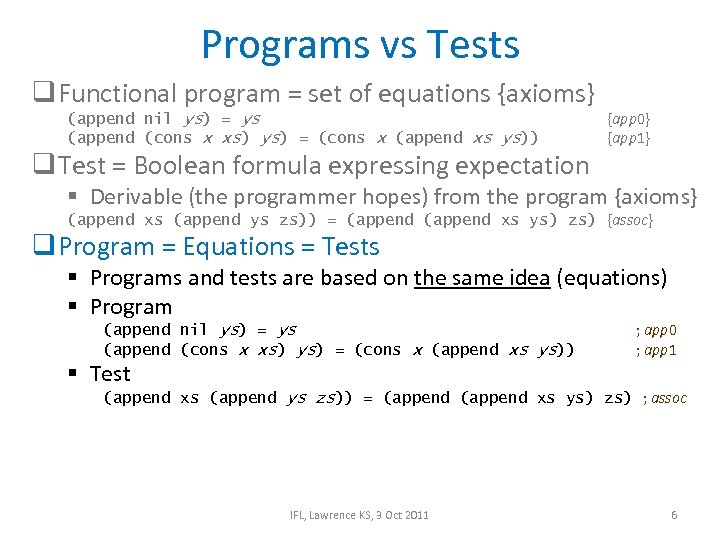Programs vs Tests q Functional program = set of equations {axioms} (append nil ys) = ys (append (cons x xs) ys) = (cons x (append xs ys)) q Test = Boolean formula expressing expectation {app 0} {app 1} § Derivable (the programmer hopes) from the program {axioms} (append xs (append ys zs)) = (append xs ys) zs) {assoc} q Program = Equations = Tests § Programs and tests are based on the same idea (equations) § Program (append nil ys) = ys (append (cons x xs) ys) = (cons x (append xs ys)) § Test ; app 0 ; app 1 (append xs (append ys zs)) = (append xs ys) zs) ; assoc IFL, Lawrence KS, 3 Oct 2011 6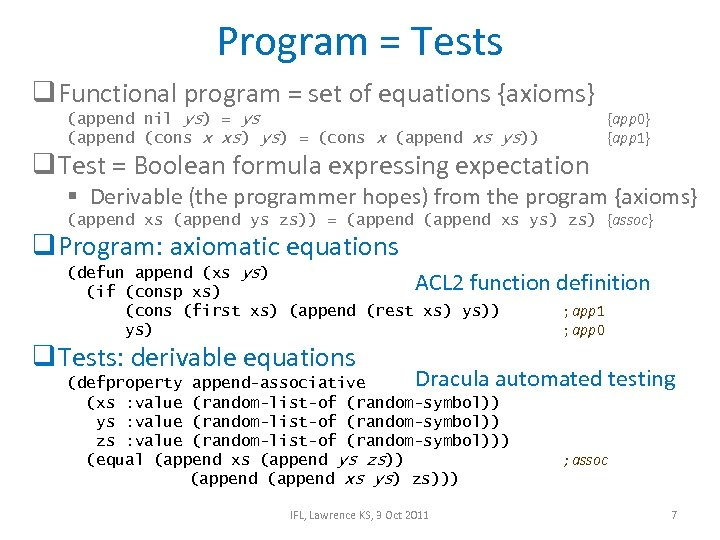Program = Tests q Functional program = set of equations {axioms} (append nil ys) = ys (append (cons x xs) ys) = (cons x (append xs ys)) q Test = Boolean formula expressing expectation {app 0} {app 1} § Derivable (the programmer hopes) from the program {axioms} (append xs (append ys zs)) = (append xs ys) zs) {assoc} q Program: axiomatic equations (defun append (xs ys) ACL 2 function definition (if (consp xs) (cons (first xs) (append (rest xs) ys)) ; app 1 ys) ; app 0 q Tests: derivable equations Dracula automated testing (defproperty append-associative (xs : value (random-list-of (random-symbol)) ys : value (random-list-of (random-symbol)) zs : value (random-list-of (random-symbol))) (equal (append xs (append ys zs)) ; assoc (append xs ys) zs))) IFL, Lawrence KS, 3 Oct 2011 7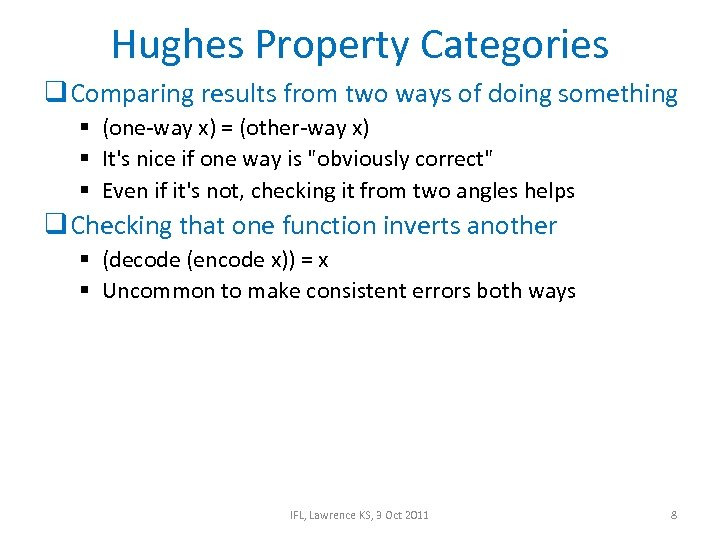Hughes Property Categories q Comparing results from two ways of doing something § (one-way x) = (other-way x) § It's nice if one way is "obviously correct" § Even if it's not, checking it from two angles helps q Checking that one function inverts another § (decode (encode x)) = x § Uncommon to make consistent errors both ways IFL, Lawrence KS, 3 Oct 2011 8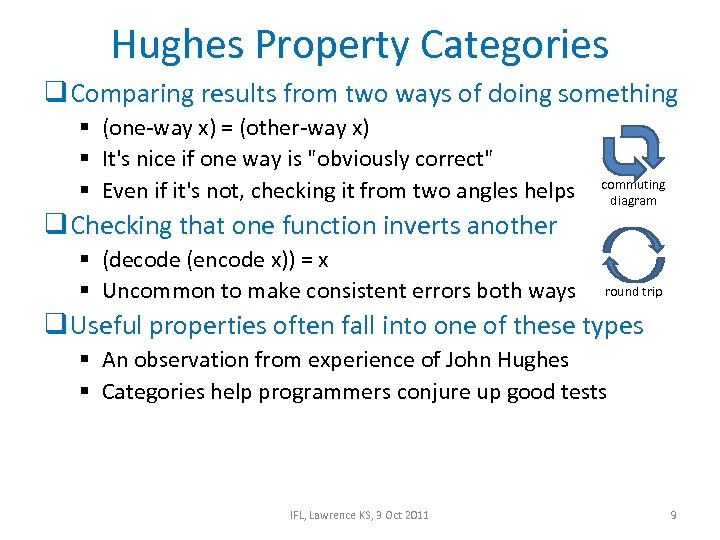Hughes Property Categories q Comparing results from two ways of doing something § (one-way x) = (other-way x) § It's nice if one way is "obviously correct" § Even if it's not, checking it from two angles helps q Checking that one function inverts another § (decode (encode x)) = x § Uncommon to make consistent errors both ways commuting diagram round trip q Useful properties often fall into one of these types § An observation from experience of John Hughes § Categories help programmers conjure up good tests IFL, Lawrence KS, 3 Oct 2011 9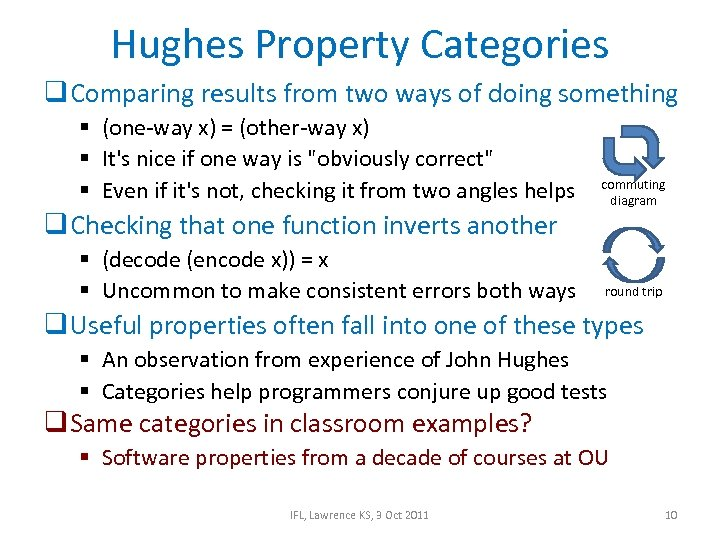Hughes Property Categories q Comparing results from two ways of doing something § (one-way x) = (other-way x) § It's nice if one way is "obviously correct" § Even if it's not, checking it from two angles helps q Checking that one function inverts another § (decode (encode x)) = x § Uncommon to make consistent errors both ways commuting diagram round trip q Useful properties often fall into one of these types § An observation from experience of John Hughes § Categories help programmers conjure up good tests q Same categories in classroom examples? § Software properties from a decade of courses at OU IFL, Lawrence KS, 3 Oct 2011 10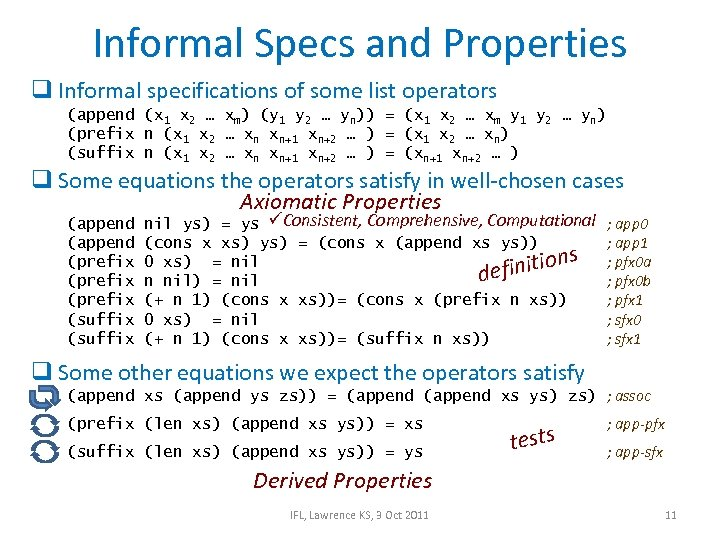Informal Specs and Properties q Informal specifications of some list operators (append (x 1 x 2 … xm) (y 1 y 2 … yn)) = (x 1 x 2 … xm y 1 y 2 … yn) (prefix n (x 1 x 2 … xn xn+1 xn+2 … ) = (x 1 x 2 … xn) (suffix n (x 1 x 2 … xn xn+1 xn+2 … ) = (xn+1 xn+2 … ) q Some equations the operators satisfy in well-chosen cases Axiomatic Properties (append (prefix (suffix nil ys) = ys üConsistent, Comprehensive, Computational (cons x xs) ys) = (cons x (append xs ys)) s 0 xs) = nil inition def n nil) = nil (+ n 1) (cons x xs))= (cons x (prefix n xs)) 0 xs) = nil (+ n 1) (cons x xs))= (suffix n xs)) ; app 0 ; app 1 ; pfx 0 a ; pfx 0 b ; pfx 1 ; sfx 0 ; sfx 1 q Some other equations we expect the operators satisfy (append xs (append ys zs)) = (append xs ys) zs) ; assoc (prefix (len xs) (append xs ys)) = xs (suffix (len xs) (append xs ys)) = ys tests ; app-pfx ; app-sfx Derived Properties IFL, Lawrence KS, 3 Oct 2011 11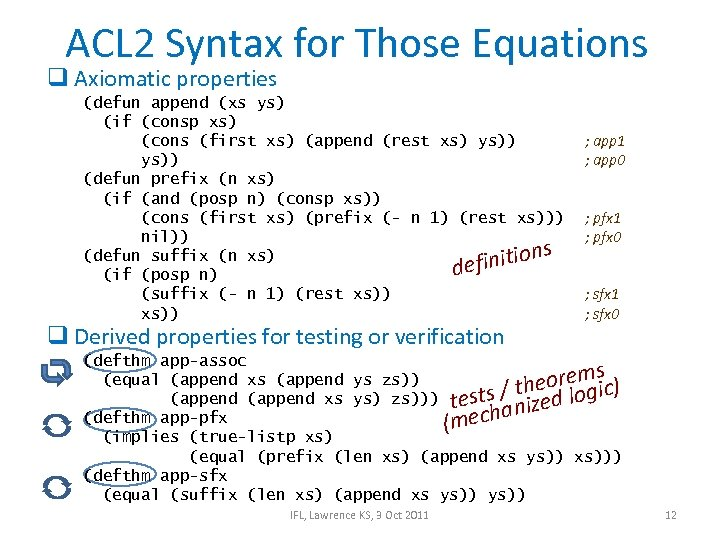ACL 2 Syntax for Those Equations q Axiomatic properties (defun append (xs ys) (if (consp xs) (cons (first xs) (append (rest xs) ys)) (defun prefix (n xs) (if (and (posp n) (consp xs)) (cons (first xs) (prefix (- n 1) (rest xs))) nil)) s (defun suffix (n xs) finition de (if (posp n) (suffix (- n 1) (rest xs)) q Derived properties for testing or verification ; app 1 ; app 0 ; pfx 1 ; pfx 0 ; sfx 1 ; sfx 0 (defthm app-assoc s (equal (append xs (append ys zs)) eorem ic) th (append xs ys) zs))) tests / zed log i (defthm app-pfx echan (m (implies (true-listp xs) (equal (prefix (len xs) (append xs ys)) xs))) (defthm app-sfx (equal (suffix (len xs) (append xs ys)) IFL, Lawrence KS, 3 Oct 2011 12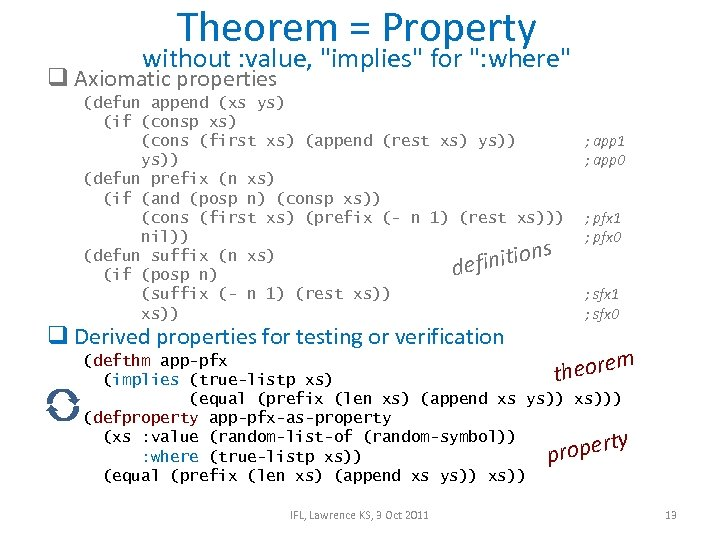Theorem = Property without : value, "implies" for ": where" q Axiomatic properties (defun append (xs ys) (if (consp xs) (cons (first xs) (append (rest xs) ys)) (defun prefix (n xs) (if (and (posp n) (consp xs)) (cons (first xs) (prefix (- n 1) (rest xs))) nil)) s (defun suffix (n xs) finition de (if (posp n) (suffix (- n 1) (rest xs)) q Derived properties for testing or verification ; app 1 ; app 0 ; pfx 1 ; pfx 0 ; sfx 1 ; sfx 0 (defthm app-pfx m theore (implies (true-listp xs) (equal (prefix (len xs) (append xs ys)) xs))) (defproperty app-pfx-as-property (xs : value (random-list-of (random-symbol)) y ropert : where (true-listp xs)) p (equal (prefix (len xs) (append xs ys)) xs)) IFL, Lawrence KS, 3 Oct 2011 13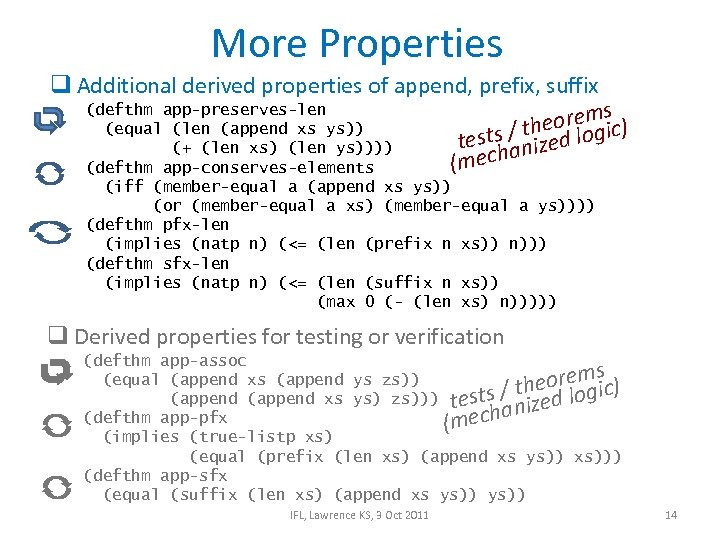More Properties q Additional derived properties of append, prefix, suffix (defthm app-preserves-len s eorem ic) (equal (len (append xs ys)) th ests / nized log t (+ (len xs) (len ys)))) a (mech (defthm app-conserves-elements (iff (member-equal a (append xs ys)) (or (member-equal a xs) (member-equal a ys)))) (defthm pfx-len (implies (natp n) (<= (len (prefix n xs)) n))) (defthm sfx-len (implies (natp n) (<= (len (suffix n xs)) (max 0 (- (len xs) n))))) q Derived properties for testing or verification (defthm app-assoc s (equal (append xs (append ys zs)) eorem ic) th (append xs ys) zs))) tests / zed log i (defthm app-pfx echan (m (implies (true-listp xs) (equal (prefix (len xs) (append xs ys)) xs))) (defthm app-sfx (equal (suffix (len xs) (append xs ys)) IFL, Lawrence KS, 3 Oct 2011 14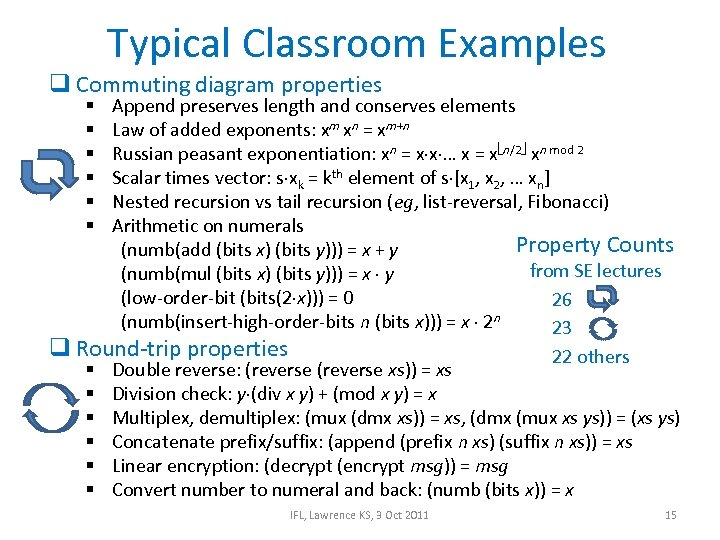Typical Classroom Examples q Commuting diagram properties Append preserves length and conserves elements Law of added exponents: xm xn = xm+n Russian peasant exponentiation: xn = x x … x = x n/2 xn mod 2 Scalar times vector: s xk = kth element of s [x 1, x 2, … xn] Nested recursion vs tail recursion (eg, list-reversal, Fibonacci) Arithmetic on numerals Property Counts (numb(add (bits x) (bits y))) = x + y from SE lectures (numb(mul (bits x) (bits y))) = x y (low-order-bit (bits(2 x))) = 0 26 (numb(insert-high-order-bits n (bits x))) = x 2 n 23 q Round-trip properties 22 others § Double reverse: (reverse xs)) = xs § Division check: y (div x y) + (mod x y) = x § Multiplex, demultiplex: (mux (dmx xs)) = xs, (dmx (mux xs ys)) = (xs ys) § Concatenate prefix/suffix: (append (prefix n xs) (suffix n xs)) = xs § Linear encryption: (decrypt (encrypt msg)) = msg § Convert number to numeral and back: (numb (bits x)) = x § § § IFL, Lawrence KS, 3 Oct 2011 15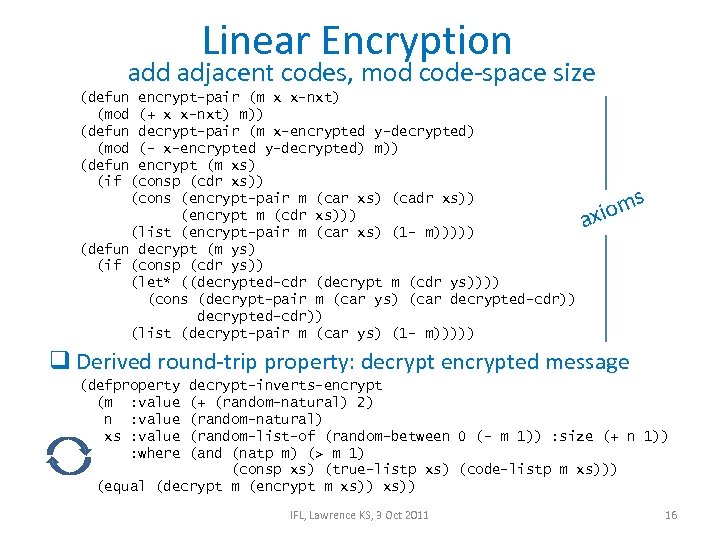Linear Encryption add adjacent codes, mod code-space size (defun encrypt-pair (m x x-nxt) (mod (+ x x-nxt) m)) (defun decrypt-pair (m x-encrypted y-decrypted) (mod (- x-encrypted y-decrypted) m)) (defun encrypt (m xs) (if (consp (cdr xs)) (cons (encrypt-pair m (car xs) (cadr xs)) (encrypt m (cdr xs))) (list (encrypt-pair m (car xs) (1 - m))))) (defun decrypt (m ys) (if (consp (cdr ys)) (let* ((decrypted-cdr (decrypt m (cdr ys)))) (cons (decrypt-pair m (car ys) (car decrypted-cdr)) (list (decrypt-pair m (car ys) (1 - m))))) xiom a s q Derived round-trip property: decrypt encrypted message (defproperty (m : value n : value xs : value : where decrypt-inverts-encrypt (+ (random-natural) 2) (random-natural) (random-list-of (random-between 0 (- m 1)) : size (+ n 1)) (and (natp m) (> m 1) (consp xs) (true-listp xs) (code-listp m xs))) (equal (decrypt m (encrypt m xs)) IFL, Lawrence KS, 3 Oct 2011 16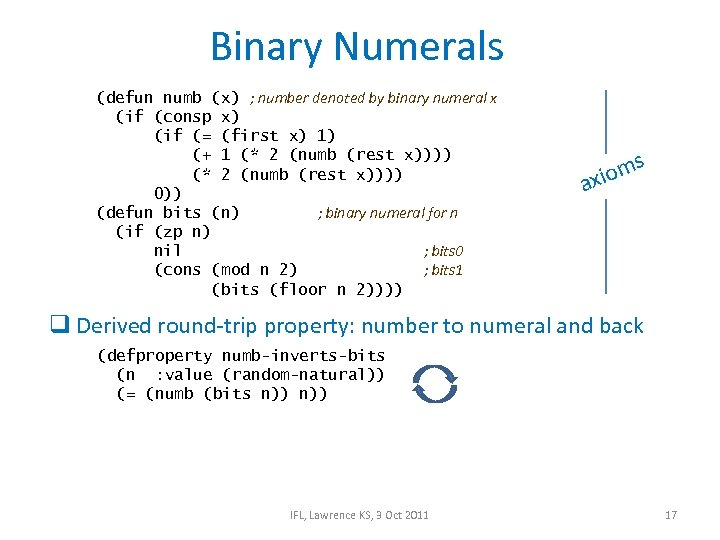Binary Numerals (defun numb (x) ; number denoted by binary numeral x (if (consp x) (if (= (first x) 1) (+ 1 (* 2 (numb (rest x)))) 0)) (defun bits (n) ; binary numeral for n (if (zp n) nil ; bits 0 (cons (mod n 2) ; bits 1 (bits (floor n 2)))) xiom a s q Derived round-trip property: number to numeral and back (defproperty numb-inverts-bits (n : value (random-natural)) (= (numb (bits n)) IFL, Lawrence KS, 3 Oct 2011 17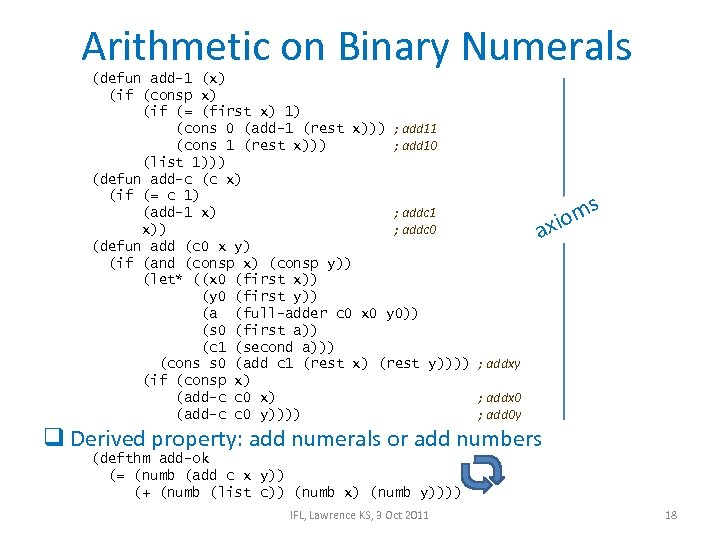Arithmetic on Binary Numerals (defun add-1 (x) (if (consp x) (if (= (first x) 1) (cons 0 (add-1 (rest x))) ; add 11 (cons 1 (rest x))) ; add 10 (list 1))) (defun add-c (c x) (if (= c 1) (add-1 x) ; addc 1 x)) ; addc 0 (defun add (c 0 x y) (if (and (consp x) (consp y)) (let* ((x 0 (first x)) (y 0 (first y)) (a (full-adder c 0 x 0 y 0)) (s 0 (first a)) (c 1 (second a))) (cons s 0 (add c 1 (rest x) (rest y)))) ; addxy (if (consp x) (add-c c 0 x) ; addx 0 (add-c c 0 y)))) ; add 0 y a m xio s q Derived property: add numerals or add numbers (defthm add-ok (= (numb (add c x y)) (+ (numb (list c)) (numb x) (numb y)))) IFL, Lawrence KS, 3 Oct 2011 18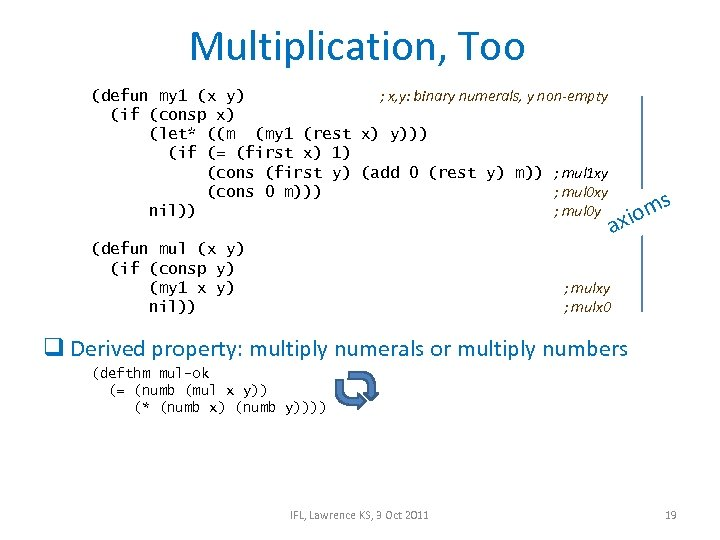Multiplication, Too (defun my 1 (x y) ; x, y: binary numerals, y non-empty (if (consp x) (let* ((m (my 1 (rest x) y))) (if (= (first x) 1) (cons (first y) (add 0 (rest y) m)) ; mul 1 xy (cons 0 m))) ; mul 0 xy nil)) ; mul 0 y (defun mul (x y) (if (consp y) (my 1 x y) nil)) ms io ax ; mulxy ; mulx 0 q Derived property: multiply numerals or multiply numbers (defthm mul-ok (= (numb (mul x y)) (* (numb x) (numb y)))) IFL, Lawrence KS, 3 Oct 2011 19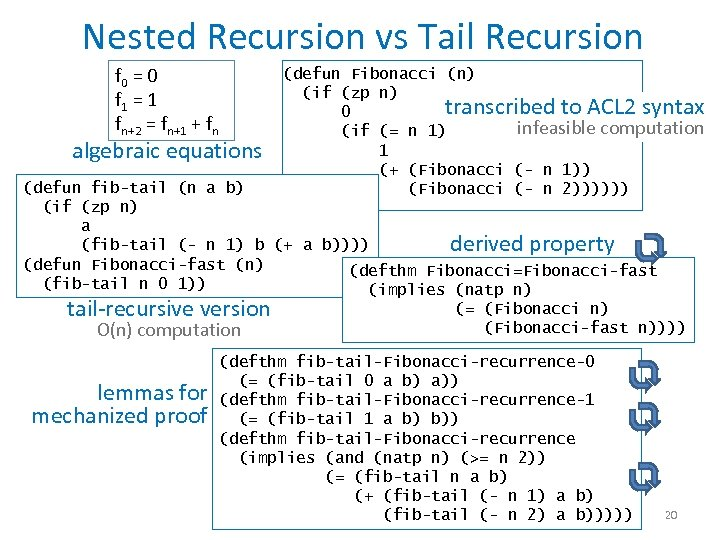Nested Recursion vs Tail Recursion f 0 = 0 f 1 = 1 fn+2 = fn+1 + fn algebraic equations (defun Fibonacci (n) (if (zp n) transcribed to ACL 2 syntax 0 infeasible computation (if (= n 1) 1 (+ (Fibonacci (- n 1)) (Fibonacci (- n 2)))))) (defun fib-tail (n a b) (if (zp n) a (fib-tail (- n 1) b (+ a b)))) derived property (defun Fibonacci-fast (n) (defthm Fibonacci=Fibonacci-fast (fib-tail n 0 1)) (implies (natp n) (= (Fibonacci n) tail-recursive version (Fibonacci-fast n)))) O(n) computation lemmas for mechanized proof (defthm fib-tail-Fibonacci-recurrence-0 (= (fib-tail 0 a b) a)) (defthm fib-tail-Fibonacci-recurrence-1 (= (fib-tail 1 a b) b)) (defthm fib-tail-Fibonacci-recurrence (implies (and (natp n) (>= n 2)) (= (fib-tail n a b) (+ (fib-tail (- n 1) a b) (fib-tail (- n 2) a b))))) 20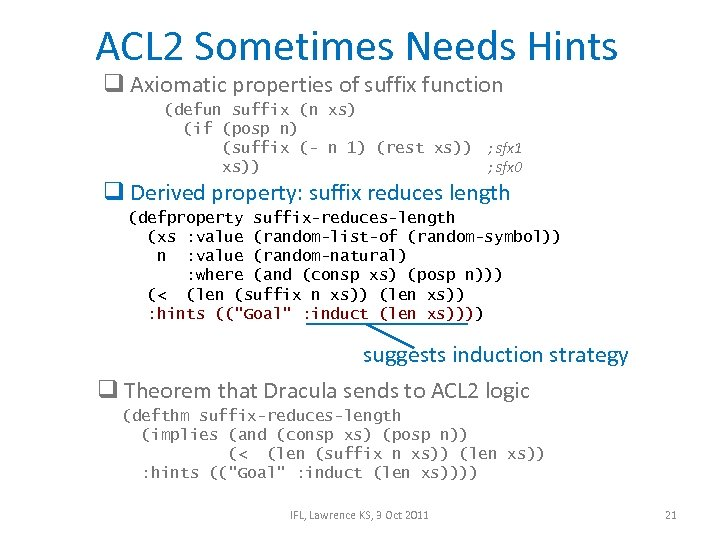ACL 2 Sometimes Needs Hints q Axiomatic properties of suffix function (defun suffix (n xs) (if (posp n) (suffix (- n 1) (rest xs)) ; sfx 1 xs)) ; sfx 0 q Derived property: suffix reduces length (defproperty suffix-reduces-length (xs : value (random-list-of (random-symbol)) n : value (random-natural) : where (and (consp xs) (posp n))) (< (len (suffix n xs)) (len xs)) : hints (("Goal" : induct (len xs)))) suggests induction strategy q Theorem that Dracula sends to ACL 2 logic (defthm suffix-reduces-length (implies (and (consp xs) (posp n)) (< (len (suffix n xs)) (len xs)) : hints (("Goal" : induct (len xs)))) IFL, Lawrence KS, 3 Oct 2011 21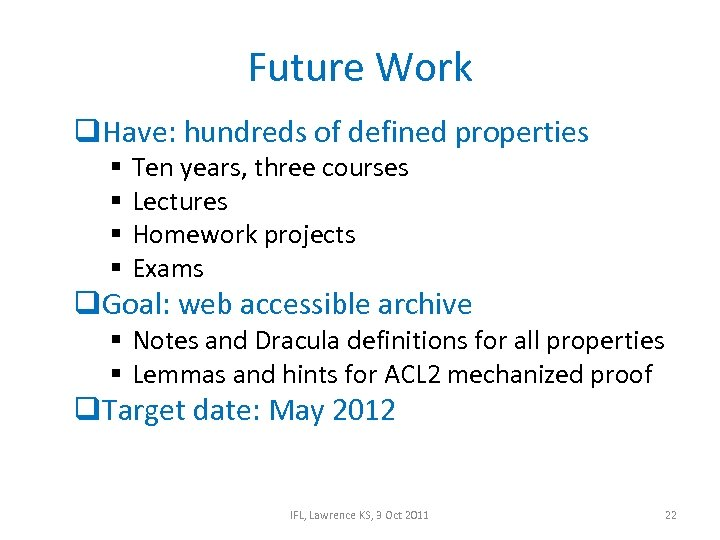Future Work q. Have: hundreds of defined properties § § Ten years, three courses Lectures Homework projects Exams q. Goal: web accessible archive § Notes and Dracula definitions for all properties § Lemmas and hints for ACL 2 mechanized proof q. Target date: May 2012 IFL, Lawrence KS, 3 Oct 2011 22The End IFL, Lawrence KS, 3 Oct 2011 23